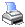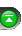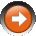﻿ Floating-Point Arithmetic
 NinjaScript > Educational Resources > Tips > Floating-Point ArithmeticSome common problems that you may encounter when comparing different double values are the caveats involved with floating-point arithmetic. Because of the way computers store floating-point numbers, under certain conditions your value will be an approximate of the actual decimal number you wanted. If this situation arises in your code, your comparison logic may not execute as you had intended even if your logic was mathematically sound on paper. To address this issue you will need to use a range comparison that takes into account the slight differences in the least significant digits of the floats.

For example, under normal mathematics we would assume double x is equivalent to double y.

 double x = 90.10; double y = 100 * 0.9010; Print(“double x: ” + x); Print(“double y: ” + y);

Even the output of this code segment suggests they are the same:

 double x = 90.1 double y = 90.1

Unfortunately, as demonstrated by this code segment, they are not.

 bool c = (x == y); Print(“x equals y: ” + c);

This segment outputs the following:

 x equals y: False

This means when we try to check for equality it would never evaluate to true even if it does mathematically.

 if (x == y)     // Do something. This will never be true.

Instead of comparing double x to y for an exact equality we will need to check a range.

 if (Math.Abs(x – y) < 0.0001)     // Do something

The arbitrary constant you choose to compare the range with should match the precision and accuracy of the floating-point numbers you are comparing.

Alternatively, you can check the difference between the two variables against the double.Epsilon field. double.Epsilon field represents the smallest possible double value.

 if (x – y < double.Epsilon)     // Do something

You can also use a Compare() method to accurately compare floating-point numbers. Take note that this method should only be used to compare price values since its precision is based on the instrument’s tick size and may be unsuited for use in other floating-point situations.

 double newPriceRange = Close - Open; double oldPriceRange = Close - Open; if (Instrument.MasterInstrument.Compare(newPriceRange, oldPriceRange) == 1) { // Do something                                 }

The Compare() method returns a value of “1” if the first parameter is greater than the second, “-1” if the first parameter is less than the second, and “0” if the first parameter is equal to the second.

For a more formal analysis of floating-point arithmetic, there are many resources online:

http://docs.sun.com/source/806-3568/ncg_goldberg.html

http://www.codeproject.com/dotnet/ExtremeFloatingPoint1.asp#terms